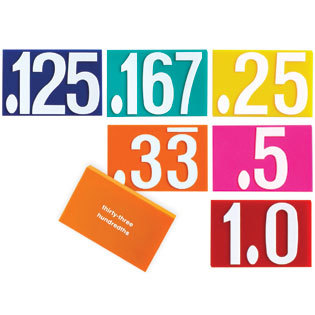Decimals
4 years ago
wmartin
Save
Edit
Host a game
Live GameLive
Homework
Solo Practice
Practice15 QuestionsShow answers
• Question 1
900 secondsQ. Name the decimal that is shown in the picture:
5.0
0.05
0.50
0.55
• Question 2
900 seconds
Q. Compare the following decimals:
6.81 ____ 6.87
<
>
=
+
• Question 3
900 secondsQ. Name the decimal that is pictured:
0.86
0.87
0.85
0.94
• Question 4
900 seconds
Q. Compare the following decimals:
6.32 ____ 6.5
<
>
+
=
• Question 5
900 secondsQ. Name the decimal that is pictured:
0.4
0.06
0.04
0.6
• Question 6
900 seconds
Q. Solve the following equation:
6.9 - 2.75 =
9.65
4.15
4.25
9.89
• Question 7
900 seconds
Q. Compare the following decimals:
6.48 ____ 6.9
<
>
+
=
• Question 8
900 seconds
Q. Compare the following decimals:
5.5 _____ 5.05
<
>
+
=
• Question 9
900 seconds
Q. Solve the following equation:
178.41 + 55.3 =
123.11
121.32
233.71
234.17
• Question 10
900 secondsQ. Name the decimal that is pictured:
14
0.014
1.4
0.14
• Question 11
900 seconds
Q. Compare the following decimals:
6.81 ____   6.9
<
>
+
=
• Question 12
900 seconds
Q. Solve the following equation:
9.12 +  2.9 =
12.02
7.17
6.22
11.41
• Question 13
900 seconds
Q. Solve the following equation:
5,321.09 - 126.8 =
5,281.29
5,194.29
5,723.02
5,447.89
• Question 14
900 secondsQ. Name the decimal that is pictured:
1.0
0.01
0.1
0.001
• Question 15
900 seconds
Q. Compare the following decimals:
3.63 ______ 3.36